Case Based Questions (MCQ)

Chapter 7 Class 12 Integrals
Serial order wise

## This change of variable formula is one of the important tools available to us in the name of integration by substitution.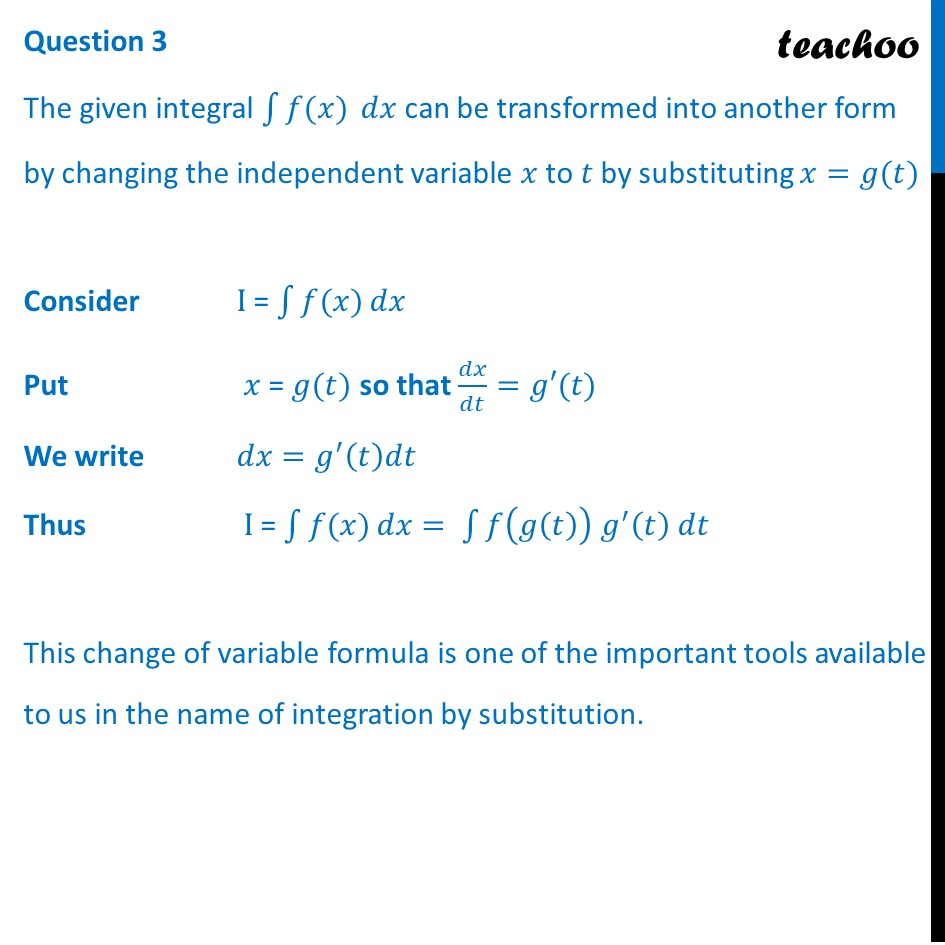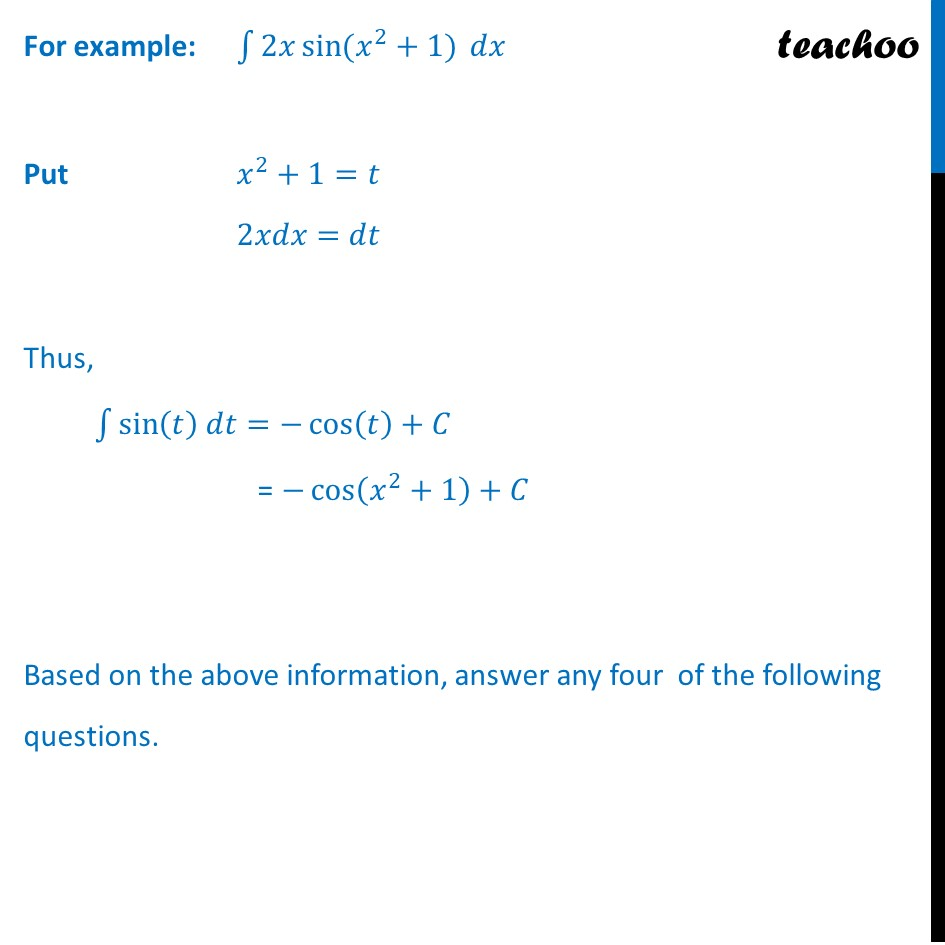## (d) None of these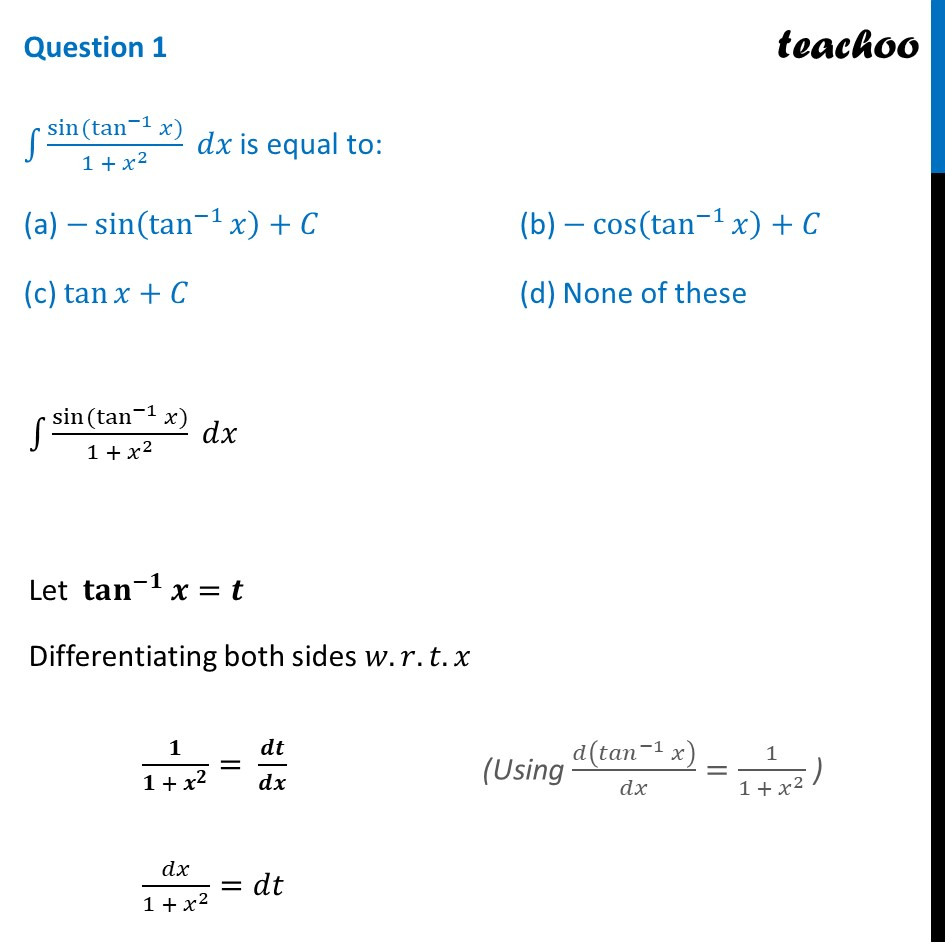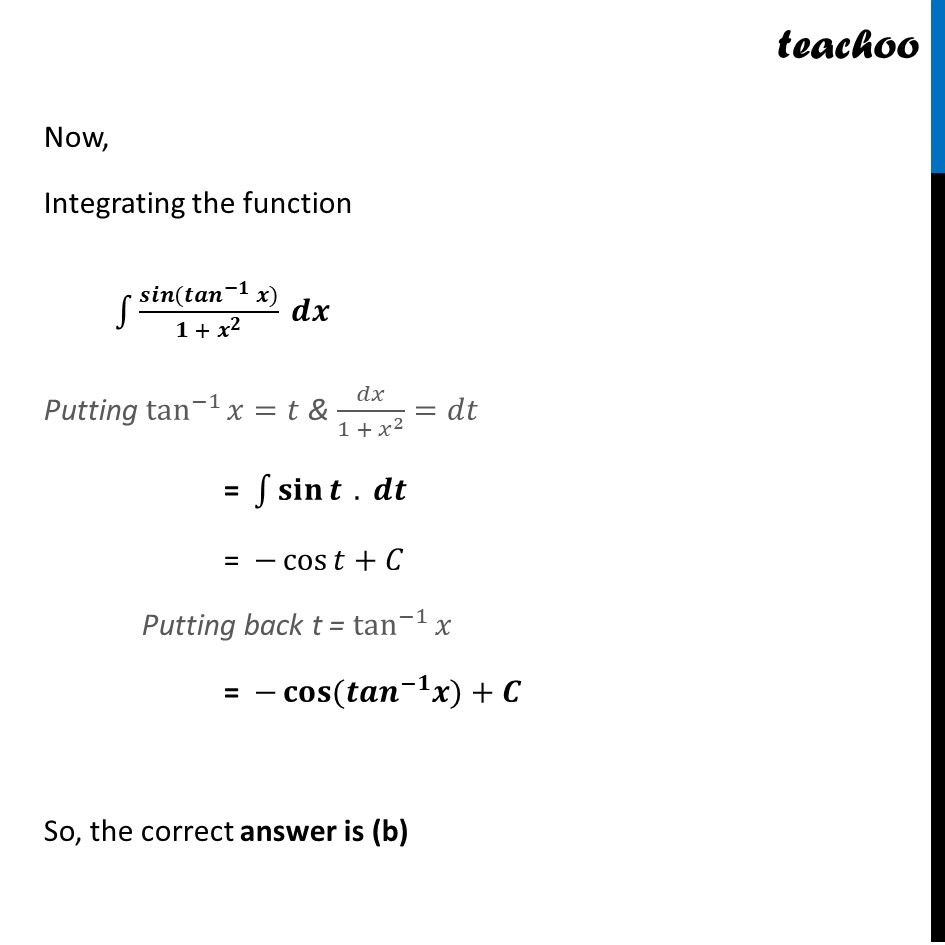## (d) None of these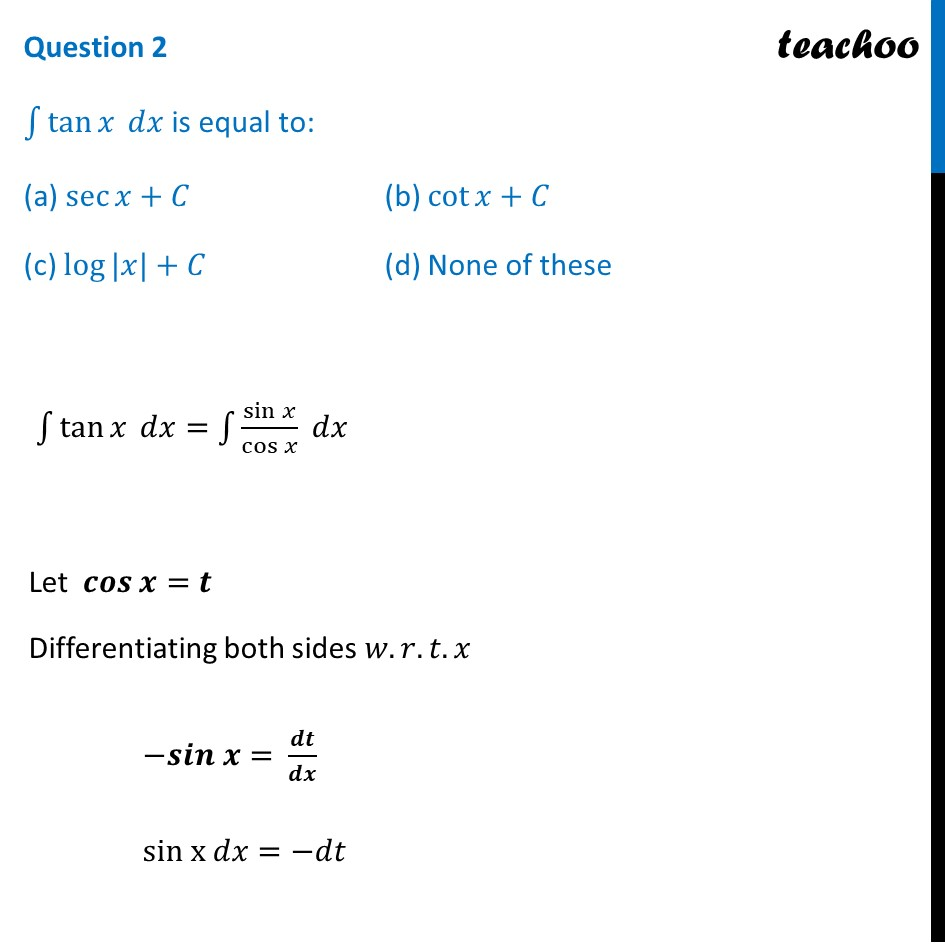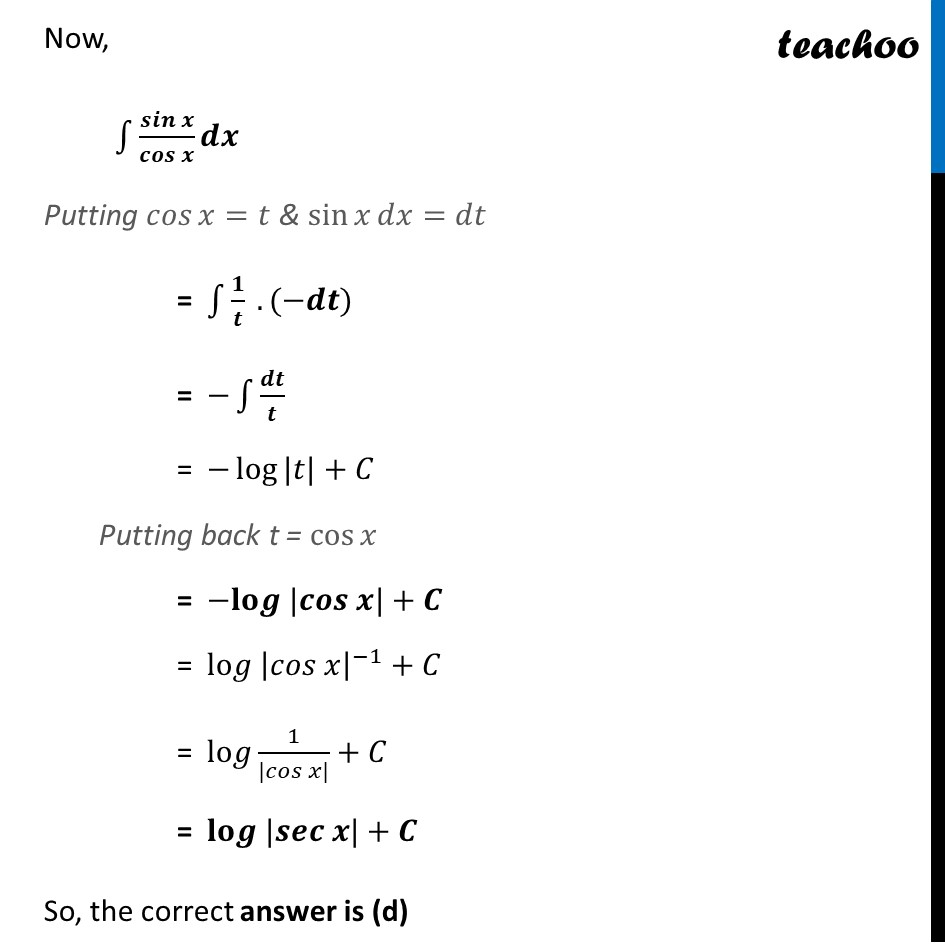## (d) None of these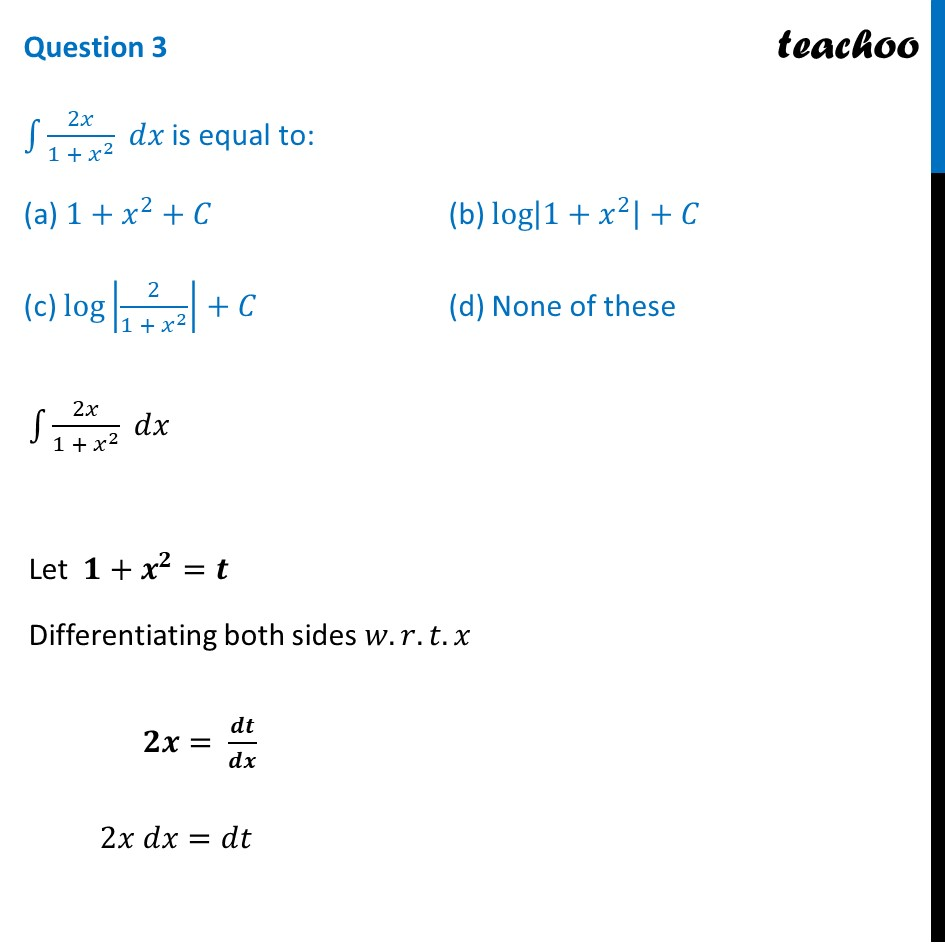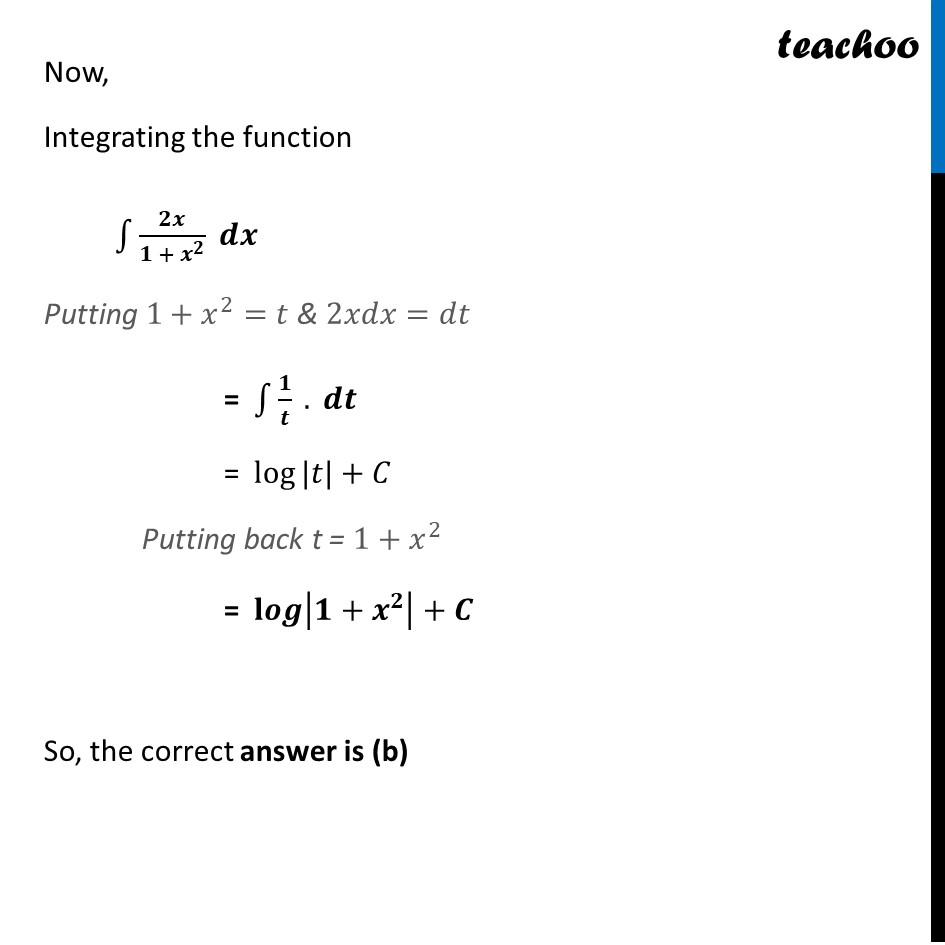## (d) None of these

This question is inspired from Ex 7.2, 5 - Chapter 7 Class 12 - Integrals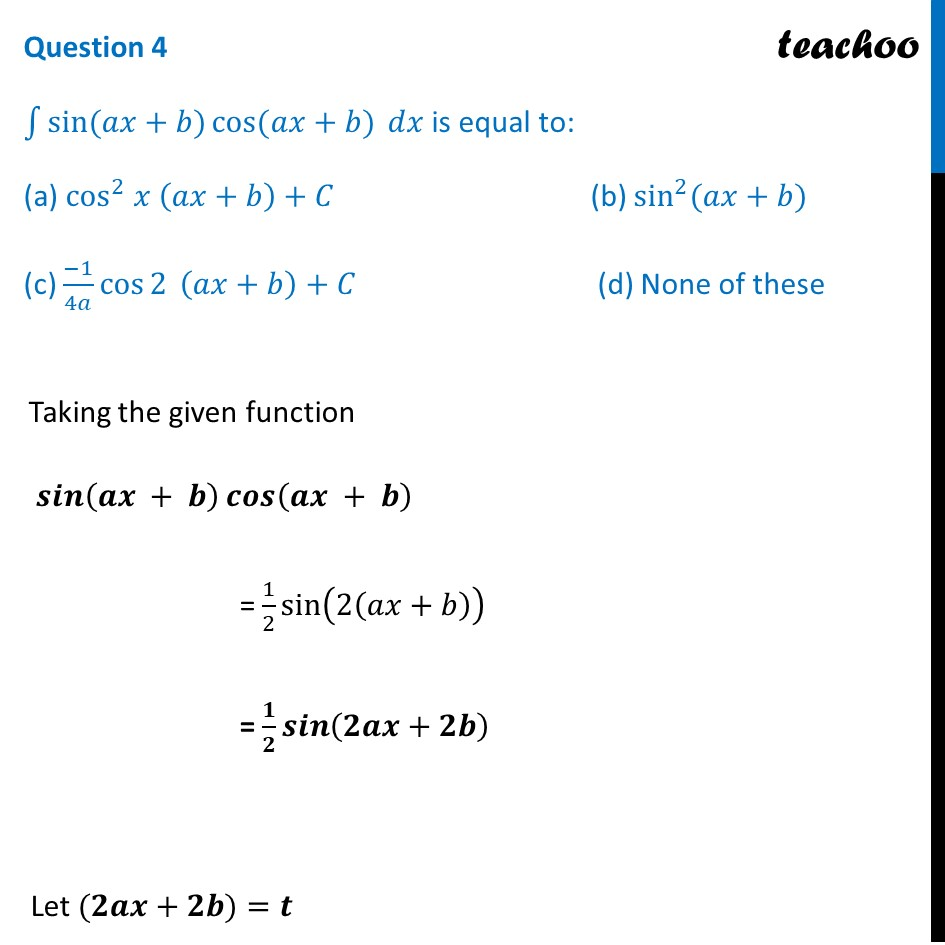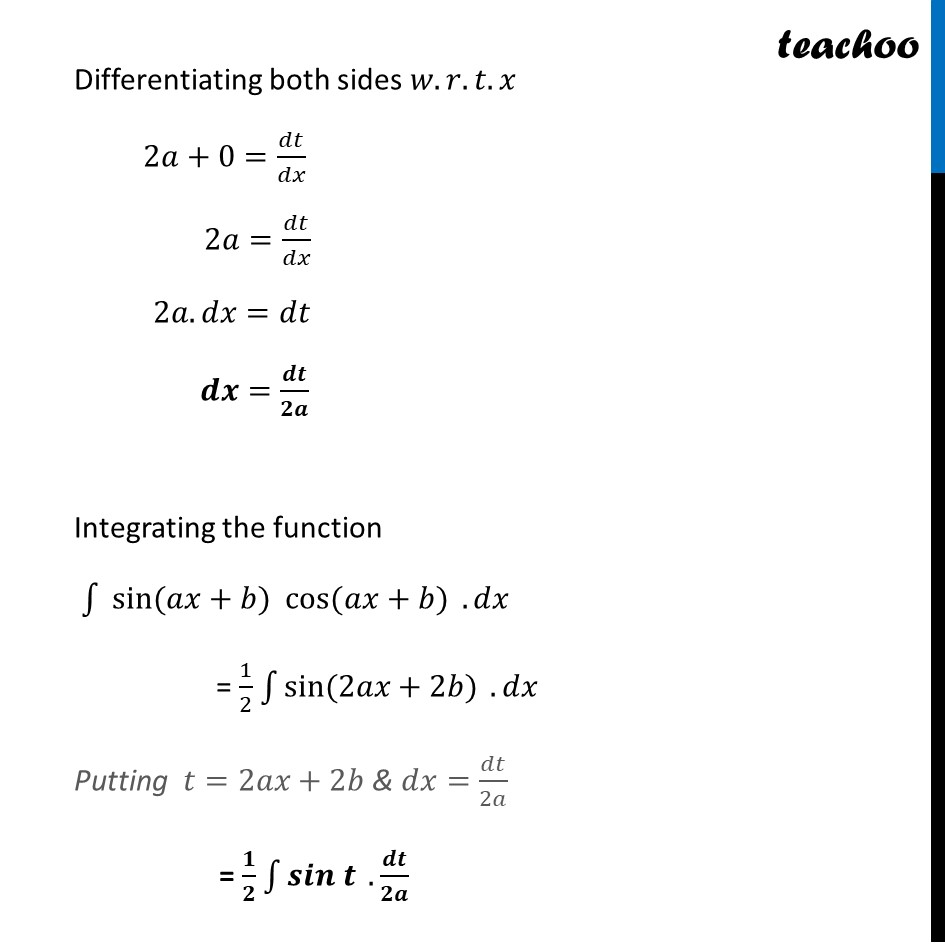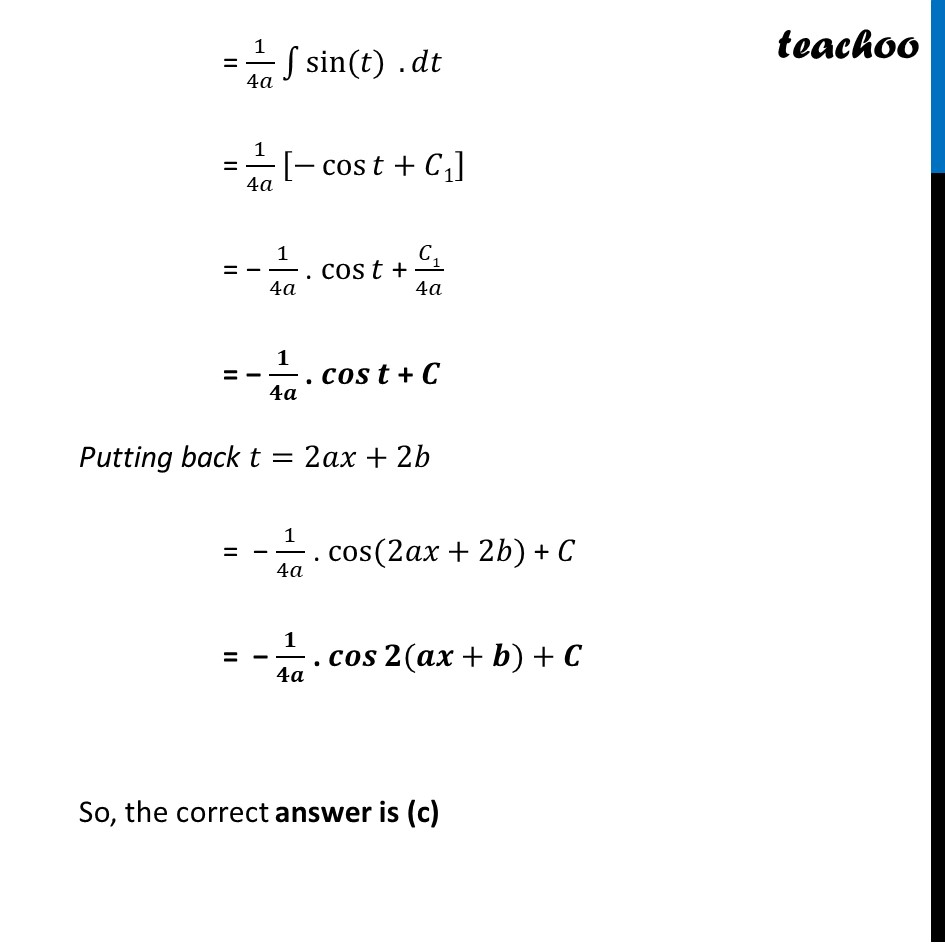## (d) None of these

This question is inspired from Ex 7.2, 3 - Chapter 7 Class 12 - Integrals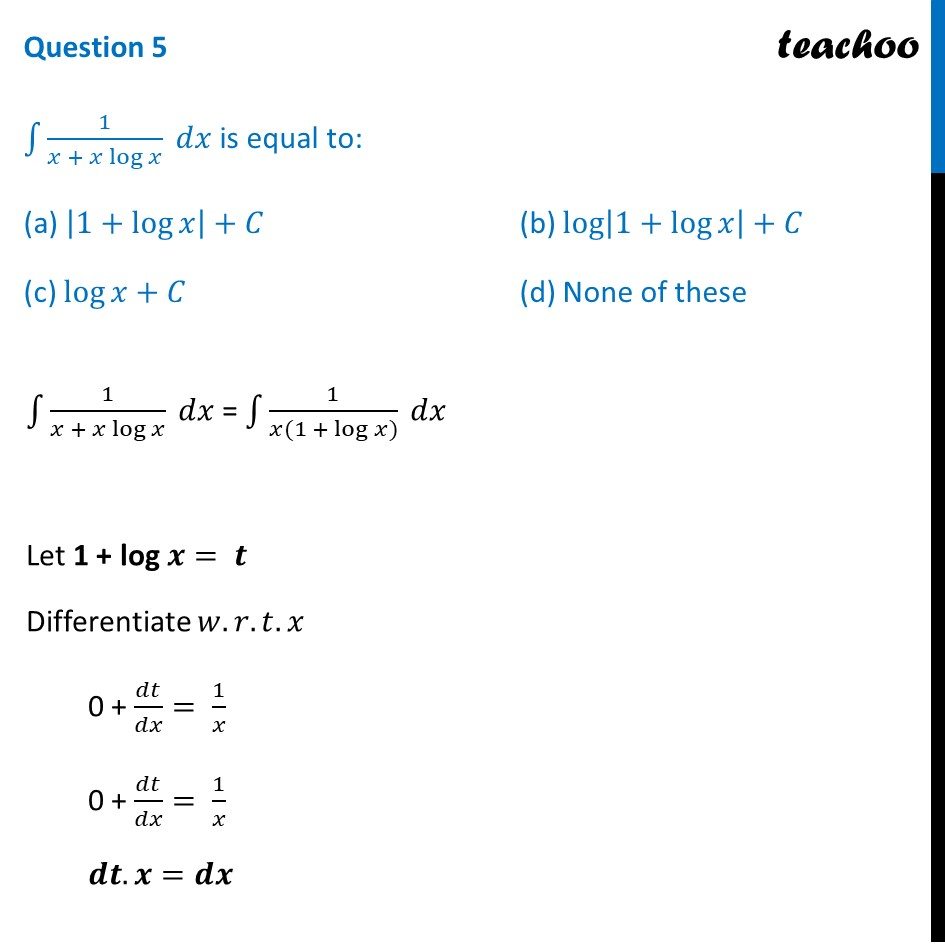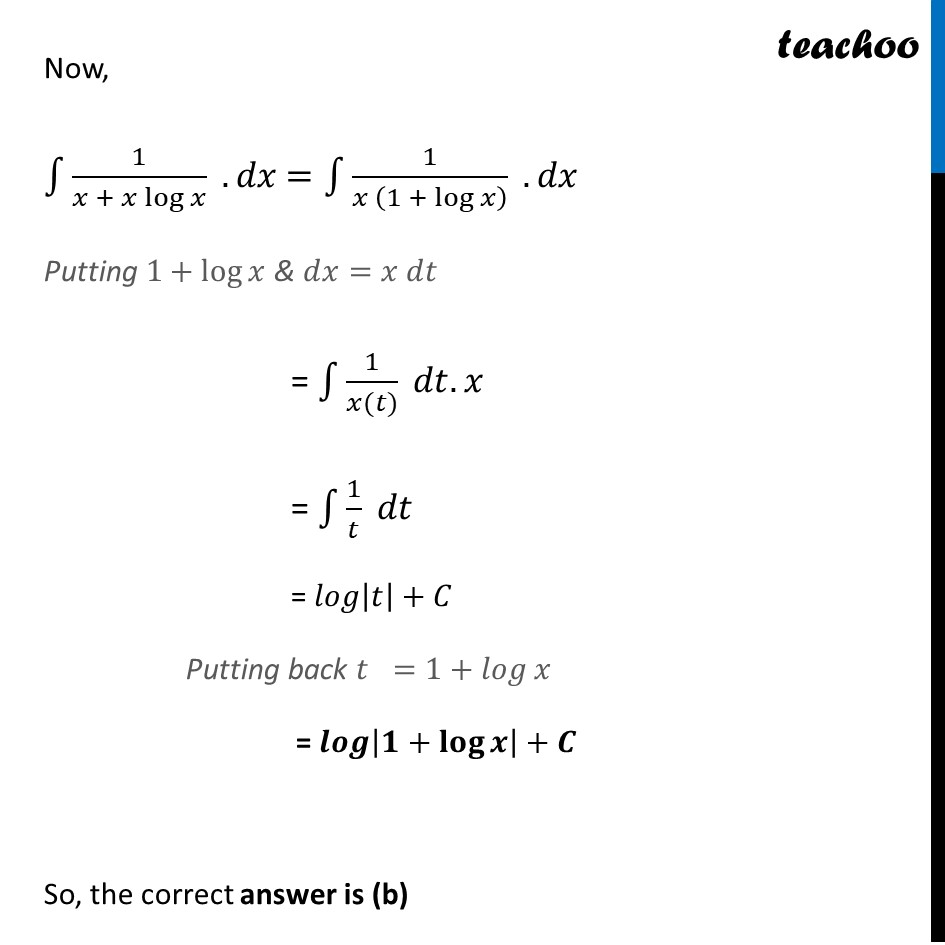Learn in your speed, with individual attention - Teachoo Maths 1-on-1 Class

### Transcript

Question 3 The given integral ∫1▒〖𝑓(𝑥)〗 𝑑𝑥 can be transformed into another form by changing the independent variable 𝑥 to 𝑡 by substituting 𝑥=𝑔(𝑡) Consider I = ∫1▒〖𝑓(𝑥)〗 𝑑𝑥 Put 𝑥 = 𝑔(𝑡) so that 𝑑𝑥/𝑑𝑡=𝑔′(𝑡) We write 𝑑𝑥=𝑔^′ (𝑡)𝑑𝑡 Thus I = ∫1▒〖𝑓(𝑥)〗 𝑑𝑥= ∫1▒〖𝑓(𝑔(𝑡)) 𝑔^′ (𝑡) 𝑑𝑡〗 This change of variable formula is one of the important tools available to us in the name of integration by substitution. For example: ∫1▒〖2𝑥 sin⁡〖(𝑥^2+1)〗 𝑑𝑥〗 Put 𝑥^2+1=𝑡 2𝑥𝑑𝑥=𝑑𝑡 Thus, ∫1▒〖sin⁡〖(𝑡) 𝑑𝑡〗=−cos⁡(𝑡)+𝐶〗 = −cos⁡〖(𝑥^2+1)+𝐶〗 Based on the above information, answer any four of the following questions. Question 1 ∫1▒〖sin⁡〖(tan^(−1)⁡𝑥)〗/(1 + 𝑥^2 ) 𝑑𝑥〗 is equal to: (a) −sin⁡(tan^(−1)⁡𝑥 )+𝐶 (b) −cos⁡(tan^(−1)⁡𝑥 )+𝐶 (c) tan⁡𝑥+𝐶 (d) None of these ∫1▒〖sin⁡〖(tan^(−1)⁡𝑥)〗/(1 + 𝑥^2 ) 𝑑𝑥〗 Let 〖𝐭𝐚𝐧〗^(−𝟏)⁡𝒙=𝒕 Differentiating both sides 𝑤.𝑟.𝑡.𝑥 𝟏/(𝟏 + 𝒙^𝟐 )= 𝒅𝒕/𝒅𝒙 𝑑𝑥/(1 + 𝑥^2 )=𝑑𝑡 Now, Integrating the function ∫1▒〖𝒔𝒊𝒏⁡〖(〖𝒕𝒂𝒏〗^(−𝟏)⁡𝒙)〗/(𝟏 + 𝒙^𝟐 ) 𝒅𝒙〗 Putting tan^(−1)⁡𝑥=𝑡 & 𝑑𝑥/(1 + 𝑥^2 )=𝑑𝑡 = ∫1▒〖𝐬𝐢𝐧⁡𝒕 . 𝒅𝒕〗 = −cos⁡𝑡+𝐶 Putting back t = tan^(−1)⁡𝑥 = −𝐜𝐨𝐬⁡〖(𝒕𝒂𝒏^(−𝟏) 𝒙)〗+𝑪 So, the correct answer is (b) Question 2 ∫1▒〖tan⁡𝑥 𝑑𝑥〗 is equal to: (a) sec⁡𝑥+𝐶 (b) cot⁡𝑥+𝐶 (c) log⁡〖|𝑥|〗+𝐶 (d) None of these ∫1▒〖tan⁡𝑥 𝑑𝑥〗=∫1▒〖sin⁡𝑥/cos⁡𝑥 𝑑𝑥〗 Let 𝒄𝒐𝒔⁡𝒙=𝒕 Differentiating both sides 𝑤.𝑟.𝑡.𝑥 −𝒔𝒊𝒏 𝒙= 𝒅𝒕/𝒅𝒙 sin x 𝑑𝑥=−𝑑𝑡 Now, ∫1▒(𝒔𝒊𝒏 𝒙)/(𝒄𝒐𝒔 𝒙) 𝒅𝒙 Putting 𝑐𝑜𝑠⁡𝑥=𝑡 & sin⁡𝑥 𝑑𝑥=𝑑𝑡 = ∫1▒〖𝟏/𝒕 . (−𝒅𝒕)〗 = −∫1▒𝒅𝒕/𝒕 = −log⁡〖|𝑡|〗+𝐶 Putting back t = cos⁡𝑥 = −𝐥𝐨𝒈 |𝒄𝒐𝒔 𝒙|+𝑪 = lo𝑔 |𝑐𝑜𝑠 𝑥|^(−1)+𝐶 = lo𝑔 1/(|𝑐𝑜𝑠 𝑥|)+𝐶 = 𝐥𝐨𝒈 |𝒔𝒆𝒄 𝒙|+𝑪 So, the correct answer is (d) Question 3 ∫1▒〖2𝑥/(1 + 𝑥^2 ) 𝑑𝑥〗 is equal to: (a) 1+𝑥^2+𝐶 (b) log⁡〖|1+𝑥^2 |+𝐶〗 (c) log⁡|2/(1 + 𝑥^2 )|+𝐶 (d) None of these ∫1▒〖2𝑥/(1 + 𝑥^2 ) 𝑑𝑥〗 Let 𝟏+𝒙^𝟐=𝒕 Differentiating both sides 𝑤.𝑟.𝑡.𝑥 𝟐𝒙= 𝒅𝒕/𝒅𝒙 2𝑥 𝑑𝑥=𝑑𝑡 Now, Integrating the function ∫1▒〖𝟐𝒙/(𝟏 + 𝒙^𝟐 ) 𝒅𝒙〗 Putting 1+𝑥^2=𝑡 & 2𝑥𝑑𝑥=𝑑𝑡 = ∫1▒〖𝟏/𝒕 . 𝒅𝒕〗 = log⁡〖|𝑡|〗+𝐶 Putting back t = 1+𝑥^2 = 𝐥𝒐𝒈|𝟏+𝒙^𝟐 |+𝑪 So, the correct answer is (b) Question 4 ∫1▒〖sin⁡〖(𝑎𝑥+𝑏)〗 cos⁡〖(𝑎𝑥+𝑏)〗 𝑑𝑥〗 is equal to: (a) cos^2 𝑥 (𝑎𝑥+𝑏)+𝐶 (b) sin^2⁡〖(𝑎𝑥+𝑏)〗 (c) (−1)/4𝑎 cos⁡2 (𝑎𝑥+𝑏)+𝐶 (d) None of these Taking the given function 𝒔𝒊𝒏⁡(𝒂𝒙 + 𝒃) 𝒄𝒐𝒔⁡(𝒂𝒙 + 𝒃) = 1/2 sin⁡(2(𝑎𝑥+𝑏)) = 𝟏/𝟐 𝒔𝒊𝒏⁡(𝟐𝒂𝒙+𝟐𝒃) Let (𝟐𝒂𝒙+𝟐𝒃)=𝒕 Differentiating both sides 𝑤.𝑟.𝑡.𝑥 2𝑎+0=𝑑𝑡/𝑑𝑥 2𝑎=𝑑𝑡/𝑑𝑥 2𝑎.𝑑𝑥=𝑑𝑡 𝒅𝒙=𝒅𝒕/𝟐𝒂 Integrating the function ∫1▒〖" " sin⁡(𝑎𝑥+𝑏) cos⁡(𝑎𝑥+𝑏)" " 〗.𝑑𝑥 = 1/2 ∫1▒〖sin⁡(2𝑎𝑥+2𝑏)〗 .𝑑𝑥 Putting 𝑡=2𝑎𝑥+2𝑏 & 𝑑𝑥=𝑑𝑡/2𝑎 = 𝟏/𝟐 ∫1▒〖𝒔𝒊𝒏 𝒕〗 .𝒅𝒕/𝟐𝒂 = 1/4𝑎 ∫1▒〖sin⁡(𝑡)〗 .𝑑𝑡 = 1/4𝑎 [−cos⁡𝑡+𝐶1] = − 1/4𝑎 . cos⁡𝑡 + 𝐶1/4𝑎 = − 𝟏/𝟒𝒂 . 𝒄𝒐𝒔⁡𝒕 + 𝑪 Putting back 𝑡=2𝑎𝑥+2𝑏 = − 1/4𝑎 . cos⁡〖(2𝑎𝑥+2𝑏)〗 + 𝐶 = − 𝟏/𝟒𝒂 . 𝒄𝒐𝒔⁡〖𝟐(𝒂𝒙+𝒃)〗+𝑪 So, the correct answer is (c) Question 5 ∫1▒〖1/(𝑥 + 𝑥 log⁡𝑥 ) 𝑑𝑥〗 is equal to: (a) |1+log⁡𝑥 |+𝐶 (b) log⁡〖|1+log⁡𝑥 |+𝐶〗 (c) log⁡𝑥+𝐶 (d) None of these ∫1▒〖1/(𝑥 + 𝑥 log⁡𝑥 ) 𝑑𝑥〗 = ∫1▒〖1/(𝑥(1 + log⁡𝑥)) 𝑑𝑥〗 Let 1 + log 𝒙= 𝒕 Differentiate 𝑤.𝑟.𝑡.𝑥 0 + 𝑑𝑡/𝑑𝑥= 1/𝑥 0 + 𝑑𝑡/𝑑𝑥= 1/𝑥 𝒅𝒕.𝒙=𝒅𝒙 Now, ∫1▒1/(𝑥 + 𝑥 log⁡𝑥 ) .𝑑𝑥 =∫1▒1/(𝑥 (1 + log⁡𝑥 ) ) .𝑑𝑥" " Putting 1+log⁡𝑥 & 𝑑𝑥=𝑥 𝑑𝑡 = ∫1▒1/(𝑥(𝑡)) 𝑑𝑡.𝑥 = ∫1▒1/𝑡 𝑑𝑡 = 𝑙𝑜𝑔|𝑡|+𝐶 Putting back 𝑡 =1+𝑙𝑜𝑔⁡𝑥 = 𝒍𝒐𝒈|𝟏+𝐥𝐨𝐠⁡𝒙 |+𝑪 So, the correct answer is (b)